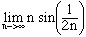Name: John Who is asking: Student Level: Secondary Question: I'm in a AP Calculus course and cannot solve the following series! And help would be greatly appreciated! Sincerely, John summation(n=1 to infinity)[n sin(1/(2n))]n Hi John, First take the absolute value of each term and then apply the root test on this series. The nth root of |n sin(1/(2n))|n is |n sin(1/(2n))| and you can evaluateby making the substitution k = 1/2n. Cheers, Harley Go to Math Central